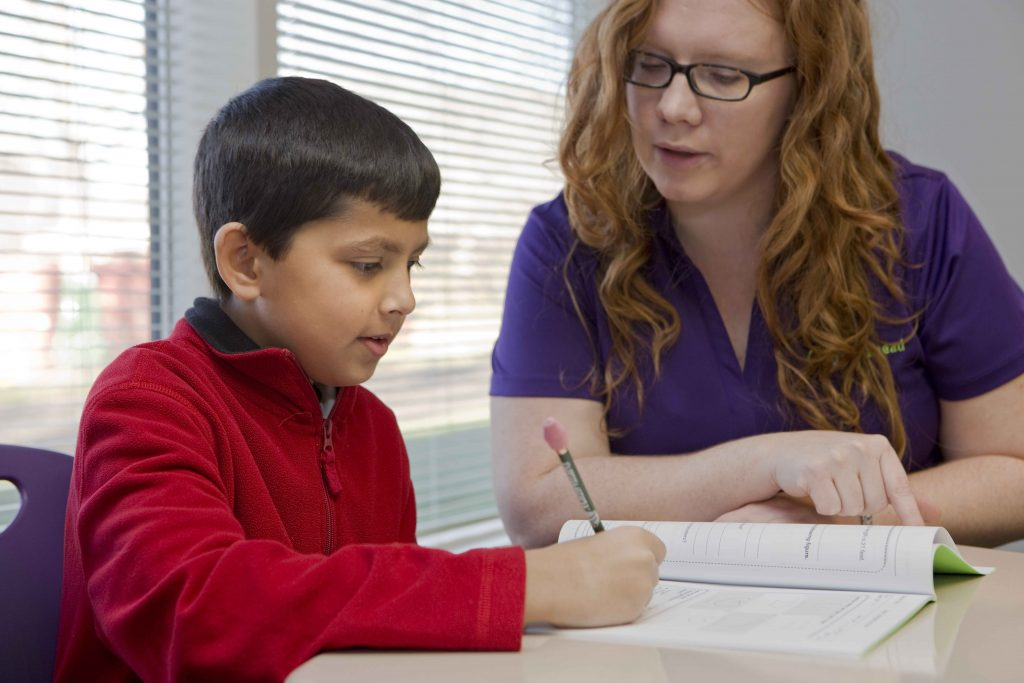# 7 Common Signs Your Child Needs Math TutoringMath is one of the most common subjects in which students struggle. It is important for struggling students to get help as soon as possible because learning math is a sequence of interdependent steps. One must fully understand the concepts of any current or previous steps before moving on to the next one. This is a common root cause for students failing to progress. Tutoring helps fill in the gaps in this sequence that students might be missing.

This is also the reason why math foundation is learned repetitively in the beginning years of students’ education: PK, KG, 1st grade, and 2nd grade and why tutoring is more common starting after 3rd grade. But how do you know if you child needs a math tutor?

# Your Child May Need Math Tutoring If…

#### 1.Your child has understood the concept of addition with carry and subtraction with borrow by the end of 2nd grade.

Addition and subtraction are learned after counting and are essential building blocks for math. They are usually taught in KG, 1st, and 2nd grades. Therefore, if your child struggles with these simple math concepts by the end of 2nd grade, he or she may need tutoring before continuing to multiplication.

#### 2. Your child has not memorized the multiplication tables by the end of 3rd grade.

Multiplication usually starts at the end of 2nd grade and continues through most of 3rd grade. Memorizing basic multiplication tables helps immensely with higher math concepts and division. If the multiplication tables are not memorized, moving on to division can be extremely challenging.

#### 3. Your child has not grasped the concept of long division by the end of 4th grade.

Long division requires multiple steps including estimation, multiplication, and subtraction. Long division is known to be one of the most common struggles in 3rd and 4th grades. The goal is for students to understand long division by the middle of 4th grade in order to continue on to fractions and decimals. Moving on to fractions and decimals before completely understanding long division is not wise.

#### 4.  Your child lacks basic conversion skills in the areas of measurement, time, and fractions to decimals or vice versa by the end of 5th grade.

Students start to learn these conversions in 1st grade.  From 1s to 5th grades, the levels of difficulty keep increasing.   The goal is to have students master the concept of conversions by the end of 5th grade before they continue on to percentages.

#### 5. Your child has not mastered the concept of PEMDAS by the end of 7th grade.

PEMDAS plays a huge role in upper-level math. It is the basic foundation that needs to be understood before continuing high-level math: Algebra 1, Geometry, and Algebra 2. PEMDAS is taught at a basic level in 3rd grade, but at a higher level in Pre-Algebra. It is a key concept to learn prior to solving equations, inequalities, etc.

#### 6. Your child does not know the Laws of Exponents by the end of 7th grade.

Laws of Exponents are the taught in Pre-Algebra. It is absolutely critical to know how to evaluate an expression involving complex exponents since that can hinder them from understanding radicals, exponent-related equations, and many other higher math concepts.

#### 7. Your child does not know how to interpret word problems.

Solving word problems is required in every grade and with every topic in math. If your child continuously struggles to comprehend and translate word problems into numbers and equations, it can be a sign that you might want to find a math tutor for your child.

What do you think? Has your child benefited by math tutoring?

Author: Elaine Timbuleng, Teacher and Writer at MathWizard, Inc.

## 3 responses to “7 Common Signs Your Child Needs Math Tutoring”

1.Braden Bills says:

My son has been having a hard time with math. Maybe I should get him a math tutor? He could definitely use the assistance!

1.admin says:

Hi, Braden! If he’s having trouble with math, it’s definitely worth looking into. We have a lot of articles with advice on whether you’re child needs tutoring, the difference in cost with the different options, and which educational program is right for your child. You can also check out aga.local to see if we offer what you’re looking for! (I hope that helps!)

Here are two I think might help:
For picking the right afterschool program
For comparing costs

2.Vinay Kumar says:

After read this article I think also my son is troubling with math and I should hire a math tutor who will teach him in the right direction.

## Subscribe

Don't miss the latest article! Stay up-to-date on our blog posts by subscribing below.